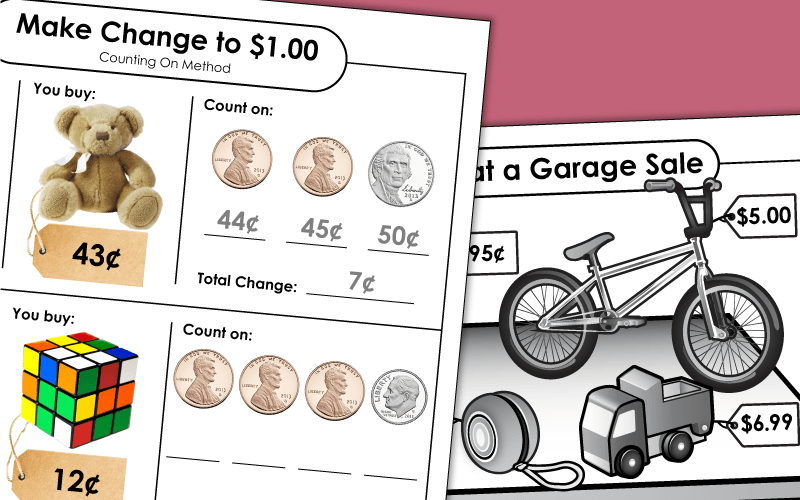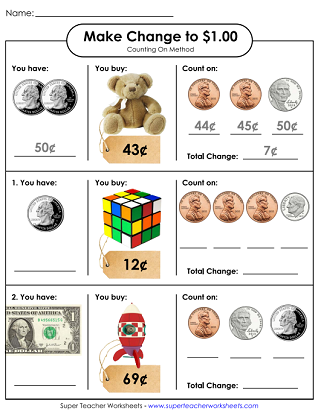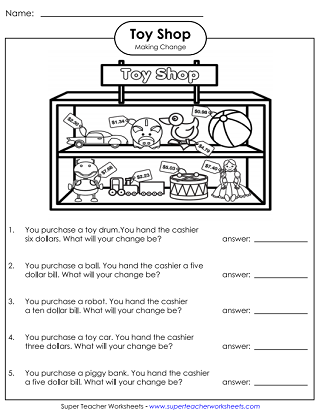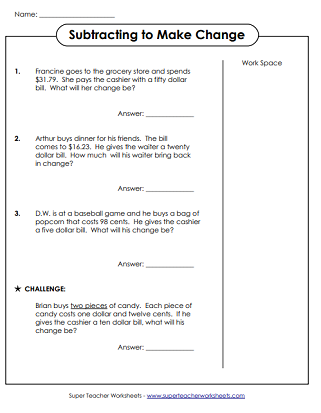# Money: Making Change

These printable worksheets and task cards can be used to supplement your lessons on making change.## Level: Basic\$1.00 and Under

Use the "counting on" method to make change for amounts up to one dollar. This graphical worksheets walks students through the process of making change step-by-step.
At the top of this worksheet is a picture of garage sale items with price tags. Below are four making change word problems.
Children must solve the word problems to make change for a dollar. An answer key is included with this basic making change worksheet.
Students will solve each of the word problems to make change for a dollar. This is a basic money worksheet for making change in amounts under one dollar. An answer key is included.
In this printable money worksheet, kids will solve the word problems to make change for a dollar. An answer key is included with this basic money skills worksheet.

Solve these graphical word problems by calculating the amount of change customers at a garage sale will receive.
Use subtraction to calculate the change given for each toy store transaction.
Use subtraction to make change. THis page includes 3 vertical problems and 3 word problems. All problems require pupils to subtract across zero.
Money Worksheets

Here you'll find worksheets on counting coins, operations with money, comparing money amounts, and rounding money.

## Sample Worksheet ImagesMy Account
Site Information